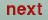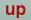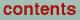Next: Plot programs Up: The STUTTGART TB-LMTO-ASA program. Previous: Frozen potential calculations

# Spin polarized calculations

A self-consistent spin-polarized calculation can be started just like a non spin-polarized (hereafter called NM) calculation, except that NSPIN=2 in Category OPTIONS and the starting moments for up and down spins in Category START are made different.

Often it is, however, faster first to perform a self-consistent NM calculation and then using this to start the spin-polarized calculation.

After the self-consistent NM calculation has been performed the atomic files contain the self-consistent moments, potential parameters and potentials. These have to be doubled (one set for each spin) and changed, before the spin-polarized iterations can begin. This can be done by changing NSPIN=1 to NSPIN=2 in Category OPTIONS and VERBOS=50 in Category IO and running lmctl.run, which inserts the doubled moments (devided by two) and potential parameters from the atomic files into the CTRL file. The potential parameters or the moments then have to be changed so that the two spin directions become different. Probably the most predictable is to change the moments. Example: Assume paramagnetic Ni has 9 d-electrons, then after lmctl.run the zeroth d moments for up and down spin are 4.5. We guess that the magnetic moment is 0.6 Bohr magnetons and the up-spin moment is changed to 4.8 and the down-spin moment is changed to 4.2. The spin polarized iterations are then started using these values in the CTRL file by setting BEGMOM=T and CNTROL=T in Category START.

The antiferromagnetic (AFM) calculation is different from the ferromagnetic and antiferrimagnetic calculations because in the latter two cases two band structure calculations have to be performed, one for spin-up and one for spin-down. In the AFM case the two band structures are identical, but the moments and potential parameters for one sublattice and say spin-up are the same as those for the other sublattice and spin-down. The program can take advantage of this by performing only one band structure calculation and interchanging the resulting moments. This is achieved by setting the token AFM=T in category OPTIONS. It should be noticed that one is not free to chose the order in which the atoms occur in the CTRL file. With the atomic order in the first sublattice chosen, the order of the second sublattice must be the same. Example: In NiO the spin-up sublattice consists of Ni-spin-up and O-spin-up and the spin-down sublattice consists of Ni-spin-down and O-spin-down. If Ni-spin-up is the first and O-spin-up the second in the CTRL file, then Ni-spin-down must be the third and O-spin-down must be the fourth atom. As described above it may be advantageous to start the spin-polarized calculations from a self-consistent NM calculation. This could be done as indicated above, but since usually there are twice as many atoms in the AFM case as in the NM case, it may be more convenient to use the program chspin.run in the main directory to produce `spin-polarized' atomic files from NM ones. To use this, just type chspin.run. It will ask for the name of the `non-spin-polarized' input file (let us call it NI) and the spin direction in the output file (U, for up or D, for down). It will produce a file with the name NIU or NID and with the same atom name in the file (NIU or NID). In this file the moments, the potential parameters, and the potentials are doubled (the moments divided by two). The potential parameters or the moments then have to be changed just as in the ferro-magnetic case described above. The extra atoms are then inserted in category SITE and CLASS BEGMOM=T and CNTROL=F in category START.Next: Plot programs Up: The STUTTGART TB-LMTO-ASA program. Previous: Frozen potential calculations

O. Jepsen
Thu Oct 12 14:48:45 MESZ 2000